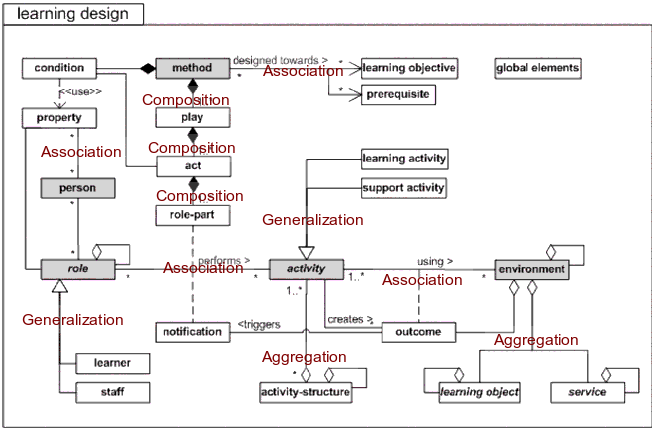# UML class diagram

Draft

## 1 Definition

UML Class diagrams are one kind of official diagram types of the Unified modeling language (UML). They describe the structure of a system.

“If someone were to come up to you in a dark alley and sy, "Psst, wanna see a UML diagram?" that diagram would probably be a class diagram.”(Fowler, 1993:35).

“A class diagram describes the types of objects in the system and the various kinds of static relationships taht exist amoung them. Class diagrams also show the properties and oprations of a class and the constraints that apply to the way objects are connected. The UML uses the term feature as a general term that covers properties and oprations of a class.” (Fowler, 1993:35).

## 2 Architecture

Class diagrams are made with quite a complex "language". Here are some elements (I know I have to go over this - Daniel K. Schneider 16:45, 5 June 2007 (MEST))

Classes
• Classes are represented with boxes
• Represented by: a rectangle with one, two or three "fields": classname, properties (attributes) and operations.
• Properties are structural elements of class. Now it get's a little bit complicated. You can describe a property either with an attribute (i.e. within the class box) or with an association (see below).
Association
• A relationship between two classes or properties
• Represented by: a solid line, or if directional with an arrow directed from the source object to the target object, or if bidirectional with an arrow on both ends.
Aggregation
• A part-of relationship
• Represented by: a solid line, with an emtpy lozange at aggregation end and arror at member end
• Example: a learning object is part of an environment
Generalization
• A is-a relationship
• Represented by: A solid line with a triangular arrow from specialized class to class
• Example: Learner is a role
Composition
• Like aggregation but you can add more constraints.
• An instance of a class can be potentially a component of several classes, but can only be owned by one.
• Represented by: a solid line, with an filled lozange at aggregation end and arror at member end
Multiplicity
• of an association end is the number of possible instances of the class associated with a single instance of the other end.
• Represented on both ends by the following syntax
MultiplicitiesExplanation

The notation n . . m indicates n to m instances.

0..1 zero or one instance.
0..*  or  * no limit on the number of instances (including none).
1 exactly one instance
1..* at least one instance
More
• There is more (sorry)...

## 3 Examples

### 3.1 IMS Learning Design

Here is the official UML Diagram that summarizes the IMS Learning Design educational modeling language:

Here is an annotated version. Blue boxes (roughly) give an idea of the relationships involved.UML diagram of IMS Learning Design annotated with UML relationships

Here is second try. Annotation is in red (blue boxes wouldn't show well enough when I pasted this into a word processor)UML diagram of IMS Learning Design annotated with UML relationships